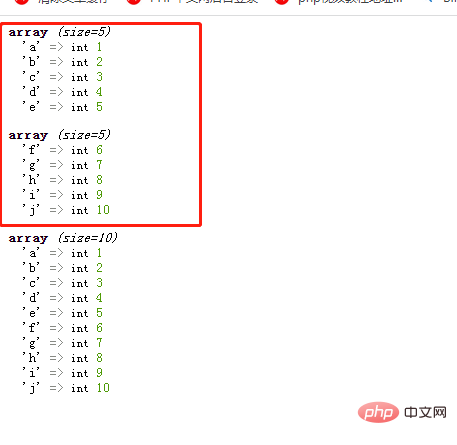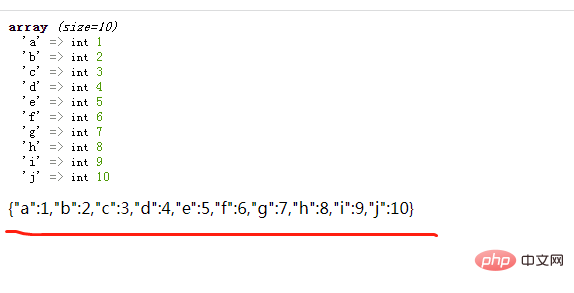# 怎么将多个php数组转成一个json数据1、使用array_merge_recursive()函数将多个数组合并为一个数组

`array_merge_recursive(array1,array2,array3...)`

```<?php
\$arr1 = array ('a'=>1,'b'=>2,'c'=>3,'d'=>4,'e'=>5);
\$arr2 = array ('f'=>6,'g'=>7,'h'=>8,'i'=>9,'j'=>10);
var_dump(\$arr1);
var_dump(\$arr2);
\$arr = array_merge_recursive(\$arr1,\$arr2);
var_dump(\$arr);
?>```2、使用json_encode()函数将合并后的数组转为json数据

json_encode()函数可以对变量进行JSON编码，返回JSON字符串。

```<?php
\$arr1 = array ('a'=>1,'b'=>2,'c'=>3,'d'=>4,'e'=>5);
\$arr2 = array ('f'=>6,'g'=>7,'h'=>8,'i'=>9,'j'=>10);
\$arr = array_merge_recursive(\$arr1,\$arr2);
var_dump(\$arr);
echo json_encode(\$arr);
?>```array_merge_recursive() 函数用于把一个或多个数组合并为一个数组。

• 该函数与 array_merge() 函数之间的不同是在处理两个或更多个数组元素有相同的键名的情况。array_merge_recursive() 不会进行键名覆盖，而是将多个相同键名的值递归组成一个数组。

json_encode()函数可以对变量进行JSON编码；成功则返回 JSON 编码的 字符串 ，失败时返回 false 。• 相关标签：php数组 php php字符串
• 相关文章

相关视频